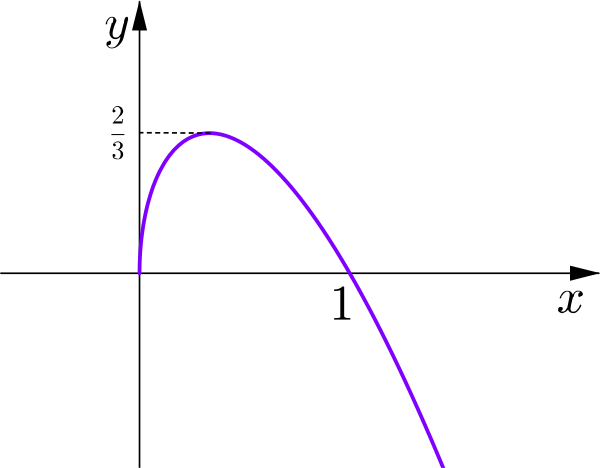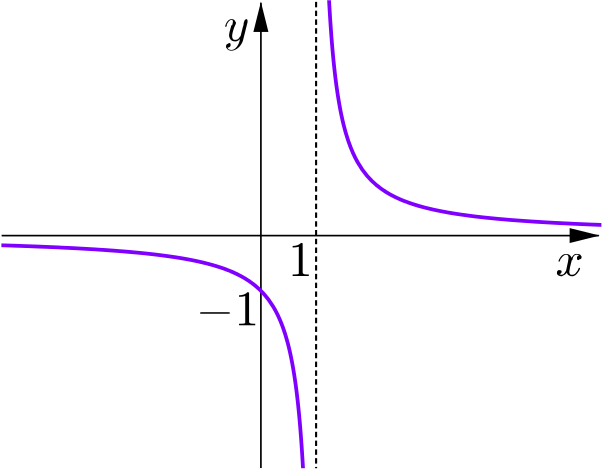### Combining Functions

Fluency exercise$f(x)=\sqrt{3x}(1-x)$$f(x)=\dfrac{1}{x-1}$